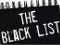# The Top 10 Things Wrong with JavaScript

`0.1 + 0.2 → 0.300000000000000040.1 + 0.2 === 0.3 → false // ???x = 1.0000000000000001x === 1 → true // ???typeof NaN → "number" // NaN is a number??? But...NaN != NaN → trueNaN !== NaN → true`
`[] + [] → "" // Empty string? These are arrays![] + {} → [object object]{} + [] → 0 // Why isn't the operation commutative???{} + {} → NaN // ???16 ==  → true // Array converted into string, then into number16 == [1,6] → false // But what is array converted into?"1,6" == [1,6] → truevar arr = [];arr.length → 0arr → "undefined" // No array bounds exception???arr = "hi";arr.length → 4 // 4??? Only one element has been added!arr["3"] → "hi" // Apparently "3" is coerced into a numberdelete(arr);arr.length → 4 // 4??? There are no elements in the array!arr → "undefined" // 7 lines above, length was "0"!var i = 1;i = i + ""; // Oops!i + 1 → "11"i - 1 → 0var j = "1";++j → 2 // Okay, but... var k = "1";k += 1 → "11" // What??? [1,5,20,10].sort() → [1, 10, 20, 5] // Why is it sorting strings???xs = ["10", "10", "10"];xs.map(parseInt) → [10, NaN, 2] // ???y = {};y[[]] = 1;Object.keys(y) → [""]`

Written by

Written by

## Richard Kenneth Eng

#### Mr. Smalltalk: https://medium.com/p/domo-arigato-mr-smalltalk-aa84e245beb9